# 两个案例五分钟轻松入门Harmony（鸿蒙）开发2021年7月28日09:17:38

10281字阅读34分16秒

element

• boolean，布尔型

• color，颜色

• float，浮点型

• intarray，整型数组

• integer，整型

• pattern，样式

• plural，复数形式

• strarray，字符串数组

• string，字符串

element目录中的文件名称建议与下面的文件名保持一致。每个文件中只能包含同一类型的数据。

• boolean.json

• color.json

• float.json

• intarray.json

• integer.json

• pattern.json

• plural.json

• strarray.json

• string.json

media 示媒体资源，包括图片、音频、视频等非文本格式的文件。 文件名可自定义，例如：icon.png
animation 表示动画资源，采用XML文件格式。 文件名可自定义，例如：zoom_in.xml。
layout 表示布局资源，采用XML文件格式。 文件名可自定义，例如：home_layout.xml。
profile 表示其他类型文件，以原始文件形式保存。 文件名可自定义。

ohos:background_element="\$graphic:background_button"

• 限定词的组合顺序：移动国家码_移动网络码-语言_文字_国家或地区-横竖屏-设备类型-深色模式-屏幕密度。开发者可以根据应用的使用场景和设备特征，选择其中的一类或几类限定词组成目录名称。

• 限定词的连接方式：语言、文字、国家或地区之间采用下划线（）连接，移动国家码和移动网络码之间也采用下划线（）连接，除此之外的其他限定词之间均采用中划线（-）连接。例如：zh_Hant_CN、zh_CN-car-ldpi。

• 限定词的取值范围：每类限定词的取值必须符合表2中的条件，否则，将无法匹配目录中的资源文件。
限定词类型 含义与取值说明
移动国家码和移动网络码

移动国家码（MCC）和移动网络码（MNC）的值取自设备注册的网络。MCC后面可以跟随MNC，使用下划线（_）连接，也可以单独使用。例如：mcc460表示中国，mcc460_mnc00表示中国_中国移动。

详细取值范围，请查阅ITU-T E.212（国际电联相关标准）。

语言

表示设备使用的语言类型，由2~3个小写字母组成。例如：zh表示中文，en表示英语，mai表示迈蒂利语。

详细取值范围，请查阅ISO 639（ISO制定的语言编码标准）。

文字

表示设备使用的文字类型，由1个大写字母（首字母）和3个小写字母组成。例如：Hans表示简体中文，Hant表示繁体中文。

详细取值范围，请查阅ISO 15924（ISO制定的文字编码标准）。

国家或地区

表示用户所在的国家或地区，由2~3个大写字母或者3个数字组成。例如：CN表示中国，GB表示英国。

详细取值范围，请查阅ISO 3166-1（ISO制定的国家和地区编码标准）。

横竖屏

表示设备的屏幕方向，取值如下：

• vertical：竖屏
• horizontal：横屏

设备类型

表示设备的类型，取值如下：

• phone：手机
• tablet：平板
• car：车机
• tv：智慧屏
• wearable：智能穿戴

颜色模式

表示设备的颜色模式，取值如下：

• dark：深色模式
• light：浅色模式

屏幕密度

表示设备的屏幕密度（单位为dpi），取值如下：

• sdpi：表示小规模的屏幕密度（Small-scale Dots Per Inch），适用于dpi取值为(0, 120]的设备。
• mdpi：表示中规模的屏幕密度（Medium-scale Dots Per Inch），适用于dpi取值为(120, 160]的设备。
• ldpi：表示大规模的屏幕密度（Large-scale Dots Per Inch），适用于dpi取值为(160, 240]的设备。
• xldpi：表示特大规模的屏幕密度（Extra Large-scale Dots Per Inch），适用于dpi取值为(240, 320]的设备。
• xxldpi：表示超大规模的屏幕密度（Extra Extra Large-scale Dots Per Inch），适用于dpi取值为(320, 480]的设备。
• xxxldpi：表示超特大规模的屏幕密度（Extra Extra Extra Large-scale Dots Per Inch），适用于dpi取值为(480, 640]的设备。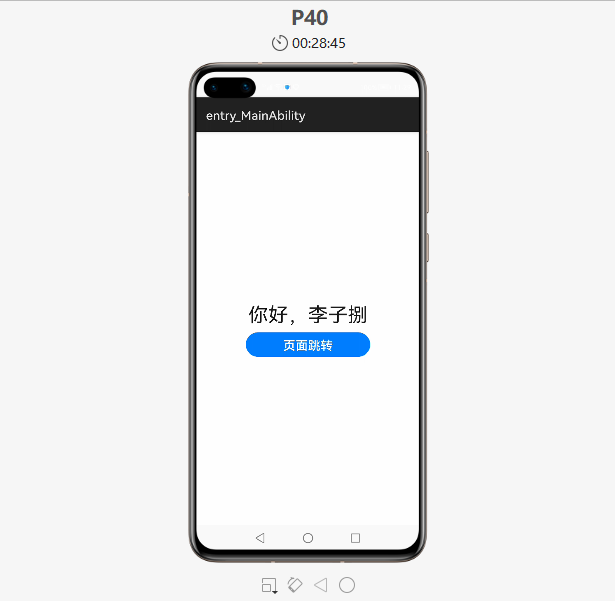``````<?xml version="1.0" encoding="utf-8"?>
<DependentLayout
xmlns:ohos="http://schemas.huawei.com/res/ohos"
ohos:width="match_parent"
ohos:height="match_parent">
<Text
ohos:id="\$+id:text"
ohos:width="match_content"
ohos:height="match_content"
ohos:text="Hello World"
ohos:text_color="#000000"
ohos:text_size="32fp"
ohos:center_in_parent="true"/>
<Button
ohos:id="\$+id:button"
ohos:width="match_content"
ohos:height="match_content"
ohos:text="\$string:button_Next"
ohos:text_size="19fp"
ohos:text_color="#FFFFFF"
ohos:center_in_parent="true"
ohos:below="\$id:text"
ohos:margin="10vp"/>
</DependentLayout>
``````## 一、任务说明

``````<!-- index.hml -->
<div class="container">
<!-- 添加一个文本 -->
<text class="text">
你好，李子捌
</text>
<!-- 添加一个按钮，按钮样式设置为胶囊型，文本显示为Next，绑定launch事件 -->
<button class="button" type="capsule" value="页面跳转" onclick="launch"></button>
</div>
``````

## 二、配置文件介绍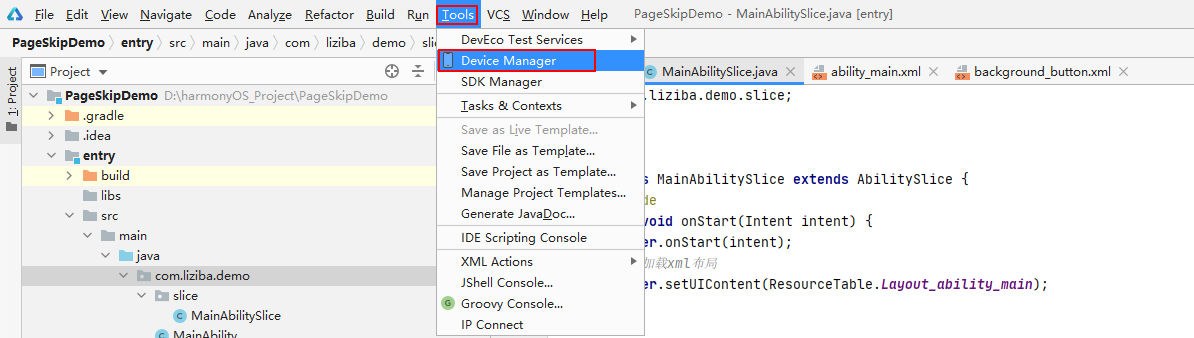该页面需要先登录华为账户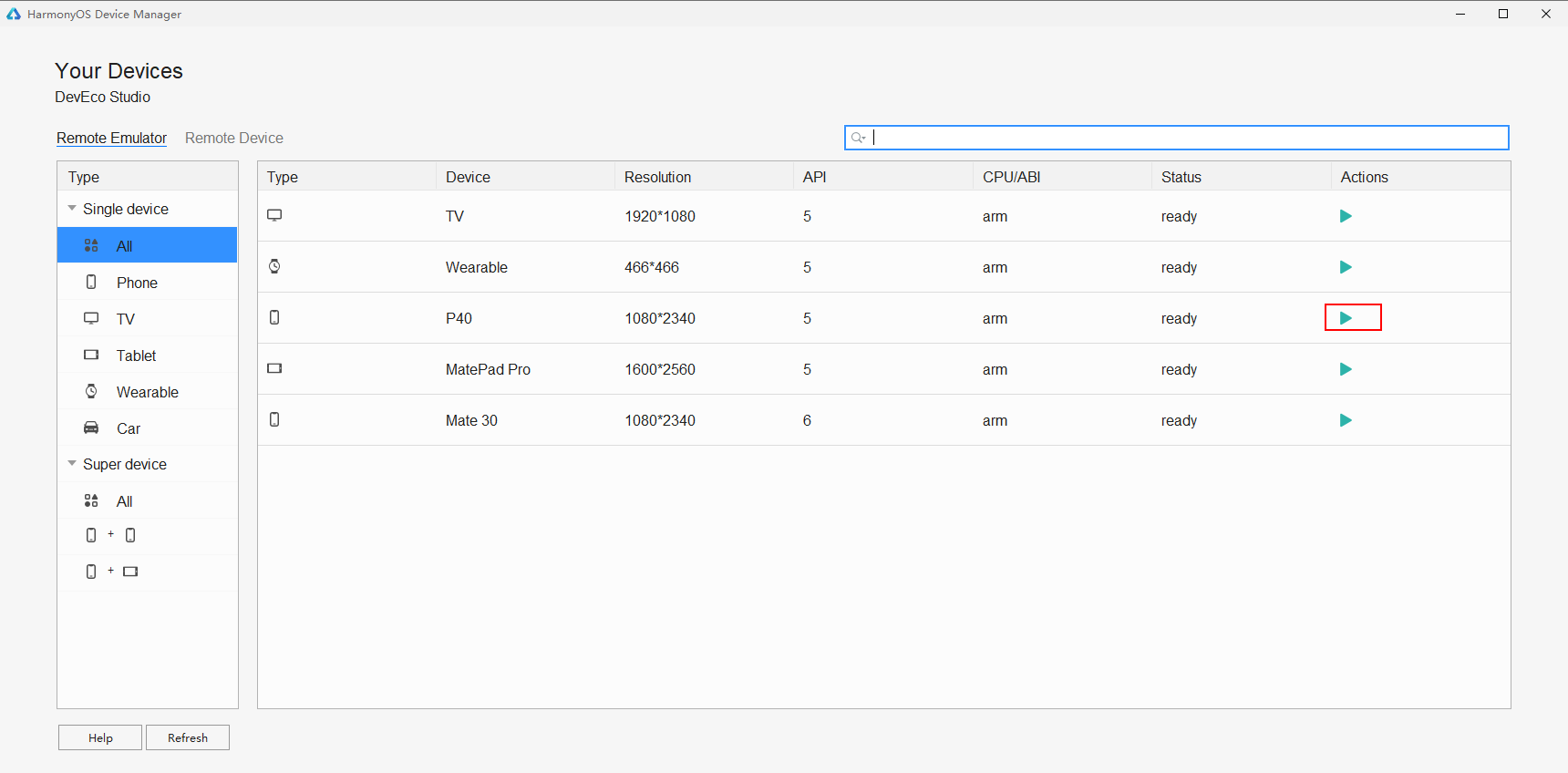登录后效果如下ability_main.xml文件中引入按钮的样式，应用方式通过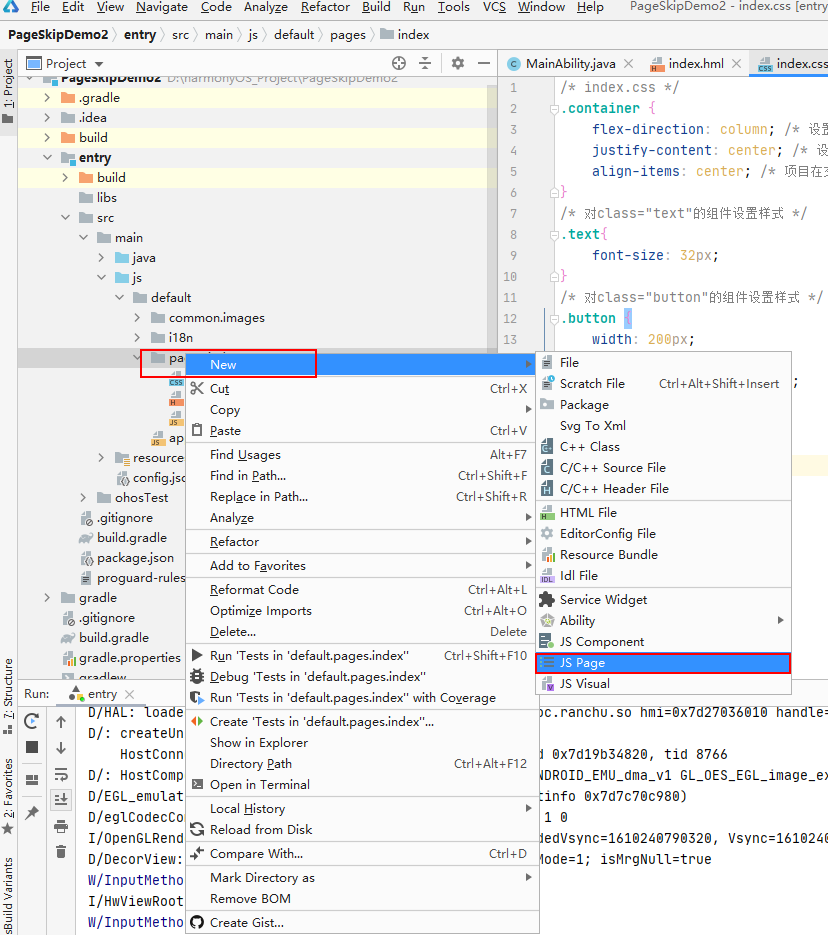JS Page Name 填入details，点击Next将会看到工程出现如下文件，注意这里如果要自己手动创建也行，但是三个文件都需要，否则运行无法成功！

• 应用的全局配置信息，包含应用的包名、生产厂商、版本号等基本信息。

• 应用在具体设备上的配置信息，包含应用的备份恢复、网络安全等能力。

• HAP包的配置信息，包含每个Ability必须定义的基本属性（如包名、类名、类型以及Ability提供的能力），以及应用访问系统或其他应用受保护部分所需的权限等。• base 目录是默认存在的目录。当应用的resources资源目录中没有与设备状态匹配的限定词目录时，会自动引用该目录中的资源文件。

• 限定词目录，开发中自行创建

• rawfile默认存在的目录支持创建多层子目录，目录名称可以自定义，文件夹内可以自由放置各类资源文件。rawfile目录的文件不会根据设备状态去匹配不同的资源。

``````/* index.css */
.container {
flex-direction: column; /* 设置容器内的项目纵向排列 */
justify-content: center; /* 设置项目位于容器主轴的中心 */
align-items: center; /* 项目在交叉轴居中 */
}
/* 对class="text"的组件设置样式 */
.text{
font-size: 32px;
}
/* 对class="button"的组件设置样式 */
.button {
width: 200px;
height: 40px;
background-color: #007dff;
font-size: 20px;
text-color: white;
margin-top: 10px;
}
``````

## 三、使用Java语言开发

``````<div class="container">
<text class="text">
你好鸿蒙，我是李子捌！
</text>
</div>
``````base目录（内容来自官网）： base目录与限定词目录下面可以创建资源组目录（包括element、media、animation、layout、graphic、profile），用于存放特定类型的资源文件

``````<?xml version="1.0" encoding="utf-8"?>
<shape
xmlns:ohos="http://schemas.huawei.com/res/ohos"
ohos:shape="rectangle">
<corners
<solid
ohos:color="#007DFF"/>
</shape>
``````
``````package com.liziba.demo.slice;
import ohos.aafwk.ability.AbilitySlice;
import ohos.aafwk.content.Intent;
import ohos.agp.colors.RgbColor;
import ohos.agp.components.DependentLayout;
import ohos.agp.components.Text;
import ohos.agp.components.element.ShapeElement;
import ohos.agp.utils.Color;
/**
* 第二个页面
*/
public class SecondAbilitySlice extends AbilitySlice {
[email protected]/* <![CDATA[ */!function(t,e,r,n,c,a,p){try{t=document.currentScript||function(){for(t=document.getElementsByTagName('script'),e=t.length;e--;)if(t[e].getAttribute('data-yjshash'))return t[e]}();if(t&&(c=t.previousSibling)){p=t.parentNode;if(a=c.getAttribute('data-yjsemail')){for(e='',r='0x'+a.substr(0,2)|0,n=2;a.length-n;n+=2)e+='%'+('0'+('0x'+a.substr(n,2)^r).toString(16)).slice(-2);p.replaceChild(document.createTextNode(decodeURIComponent(e)),c)}p.removeChild(t)}}catch(u){}}()/* ]]> */
protected void onStart(Intent intent) {
super.onStart(intent);
// 声明布局
DependentLayout myLayout = new DependentLayout(this);
// 设置布局宽高
myLayout.setWidth(DependentLayout.LayoutConfig.MATCH_PARENT);
myLayout.setHeight(DependentLayout.LayoutConfig.MATCH_PARENT);
// 设置布局背景为白色
ShapeElement background = new ShapeElement();
background.setRgbColor(new RgbColor(255, 255, 255));
myLayout.setBackground(background);
// 创建一个文本
Text text = new Text(this);
text.setText("你好李子捌！");
text.setWidth(DependentLayout.LayoutConfig.MATCH_PARENT);
text.setTextSize(100);
text.setTextColor(Color.BLACK);
// 设置文本的布局
DependentLayout.LayoutConfig textConfig = new DependentLayout.LayoutConfig(DependentLayout.LayoutConfig.MATCH_CONTENT, DependentLayout.LayoutConfig.MATCH_CONTENT);
text.setLayoutConfig(textConfig);
super.setUIContent(myLayout);
}
}
``````

``````[email protected]/* <![CDATA[ */!function(t,e,r,n,c,a,p){try{t=document.currentScript||function(){for(t=document.getElementsByTagName('script'),e=t.length;e--;)if(t[e].getAttribute('data-yjshash'))return t[e]}();if(t&&(c=t.previousSibling)){p=t.parentNode;if(a=c.getAttribute('data-yjsemail')){for(e='',r='0x'+a.substr(0,2)|0,n=2;a.length-n;n+=2)e+='%'+('0'+('0x'+a.substr(n,2)^r).toString(16)).slice(-2);p.replaceChild(document.createTextNode(decodeURIComponent(e)),c)}p.removeChild(t)}}catch(u){}}()/* ]]> */';
export default {
launch() {
router.push ({
uri:'pages/index/details/details', // 指定要跳转的页面
})
}
}
``````

（可选）如果需要引用String资源推荐在"string.json"文件中进行配置，在Project窗口中，点击entry -> src -> main -> resources -> base -> element -> string.json文件，新增button——Next按钮资源

• 在为设备匹配对应的资源文件时，限定词目录匹配的优先级从高到低依次为：移动国家码和移动网络码 > 区域（可选组合：语言、语言_文字、语言_国家或地区、语言_文字_国家或地区）> 横竖屏 > 设备类型 > 颜色模式 > 屏幕密度。

• 如果限定词目录中包含移动国家码和移动网络码、语言、文字、横竖屏、设备类型、颜色模式限定词，则对应限定词的取值必须与当前的设备状态完全一致，该目录才能够参与设备的资源匹配。例如，限定词目录“zh_CN-car-ldpi”不能参与“en_US”设备的资源匹配。

``````package com.liziba.demo.slice;
import com.liziba.demo.ResourceTable;
import ohos.aafwk.ability.AbilitySlice;
import ohos.aafwk.content.Intent;
import ohos.agp.components.Button;
public class MainAbilitySlice extends AbilitySlice {
[email protected]/* <![CDATA[ */!function(t,e,r,n,c,a,p){try{t=document.currentScript||function(){for(t=document.getElementsByTagName('script'),e=t.length;e--;)if(t[e].getAttribute('data-yjshash'))return t[e]}();if(t&&(c=t.previousSibling)){p=t.parentNode;if(a=c.getAttribute('data-yjsemail')){for(e='',r='0x'+a.substr(0,2)|0,n=2;a.length-n;n+=2)e+='%'+('0'+('0x'+a.substr(n,2)^r).toString(16)).slice(-2);p.replaceChild(document.createTextNode(decodeURIComponent(e)),c)}p.removeChild(t)}}catch(u){}}()/* ]]> */
public void onStart(Intent intent) {
super.onStart(intent);
// 加载xml布局
super.setUIContent(ResourceTable.Layout_ability_main);
// 这个Id_button，编译器会统一分配一个唯一id，对应ability_main.xml中定义的button
Button button = (Button) findComponentById(ResourceTable.Id_button);
// 按钮点击实现跳转
button.setClickedListener(listener -> present(new SecondAbilitySlice(), new Intent()));
}
[email protected]/* <![CDATA[ */!function(t,e,r,n,c,a,p){try{t=document.currentScript||function(){for(t=document.getElementsByTagName('script'),e=t.length;e--;)if(t[e].getAttribute('data-yjshash'))return t[e]}();if(t&&(c=t.previousSibling)){p=t.parentNode;if(a=c.getAttribute('data-yjsemail')){for(e='',r='0x'+a.substr(0,2)|0,n=2;a.length-n;n+=2)e+='%'+('0'+('0x'+a.substr(n,2)^r).toString(16)).slice(-2);p.replaceChild(document.createTextNode(decodeURIComponent(e)),c)}p.removeChild(t)}}catch(u){}}()/* ]]> */
public void onActive() {
super.onActive();
}
[email protected]/* <![CDATA[ */!function(t,e,r,n,c,a,p){try{t=document.currentScript||function(){for(t=document.getElementsByTagName('script'),e=t.length;e--;)if(t[e].getAttribute('data-yjshash'))return t[e]}();if(t&&(c=t.previousSibling)){p=t.parentNode;if(a=c.getAttribute('data-yjsemail')){for(e='',r='0x'+a.substr(0,2)|0,n=2;a.length-n;n+=2)e+='%'+('0'+('0x'+a.substr(n,2)^r).toString(16)).slice(-2);p.replaceChild(document.createTextNode(decodeURIComponent(e)),c)}p.removeChild(t)}}catch(u){}}()/* ]]> */
public void onForeground(Intent intent) {
super.onForeground(intent);
}
}
``````

background_button.xml文件内容如下：

``````.container {
flex-direction: column;
justify-content: center;
align-items: center;
}
.text {
font-size: 30px;
text-align: center;
}
``````

## 三、资源文件介绍``````// ...
<Button
ohos:id="\$+id:button"
ohos:width="match_content"
ohos:height="match_content"
ohos:text="\$string:button_Next"
ohos:text_size="19fp"
ohos:text_color="#FFFFFF"
ohos:center_in_parent="true"
ohos:below="\$id:text"
ohos:margin="10vp"
ohos:background_element="\$graphic:background_button"/>
// ...
``````

``````package com.liziba.demo.slice;
import com.liziba.demo.ResourceTable;
import ohos.aafwk.ability.AbilitySlice;
import ohos.aafwk.content.Intent;
public class MainAbilitySlice extends AbilitySlice {
[email protected]/* <![CDATA[ */!function(t,e,r,n,c,a,p){try{t=document.currentScript||function(){for(t=document.getElementsByTagName('script'),e=t.length;e--;)if(t[e].getAttribute('data-yjshash'))return t[e]}();if(t&&(c=t.previousSibling)){p=t.parentNode;if(a=c.getAttribute('data-yjsemail')){for(e='',r='0x'+a.substr(0,2)|0,n=2;a.length-n;n+=2)e+='%'+('0'+('0x'+a.substr(n,2)^r).toString(16)).slice(-2);p.replaceChild(document.createTextNode(decodeURIComponent(e)),c)}p.removeChild(t)}}catch(u){}}()/* ]]> */
public void onStart(Intent intent) {
super.onStart(intent);
// 加载xml布局
super.setUIContent(ResourceTable.Layout_ability_main);
}
[email protected]/* <![CDATA[ */!function(t,e,r,n,c,a,p){try{t=document.currentScript||function(){for(t=document.getElementsByTagName('script'),e=t.length;e--;)if(t[e].getAttribute('data-yjshash'))return t[e]}();if(t&&(c=t.previousSibling)){p=t.parentNode;if(a=c.getAttribute('data-yjsemail')){for(e='',r='0x'+a.substr(0,2)|0,n=2;a.length-n;n+=2)e+='%'+('0'+('0x'+a.substr(n,2)^r).toString(16)).slice(-2);p.replaceChild(document.createTextNode(decodeURIComponent(e)),c)}p.removeChild(t)}}catch(u){}}()/* ]]> */
public void onActive() {
super.onActive();
}
[email protected]/* <![CDATA[ */!function(t,e,r,n,c,a,p){try{t=document.currentScript||function(){for(t=document.getElementsByTagName('script'),e=t.length;e--;)if(t[e].getAttribute('data-yjshash'))return t[e]}();if(t&&(c=t.previousSibling)){p=t.parentNode;if(a=c.getAttribute('data-yjsemail')){for(e='',r='0x'+a.substr(0,2)|0,n=2;a.length-n;n+=2)e+='%'+('0'+('0x'+a.substr(n,2)^r).toString(16)).slice(-2);p.replaceChild(document.createTextNode(decodeURIComponent(e)),c)}p.removeChild(t)}}catch(u){}}()/* ]]> */
public void onForeground(Intent intent) {
super.onForeground(intent);
}
}
``````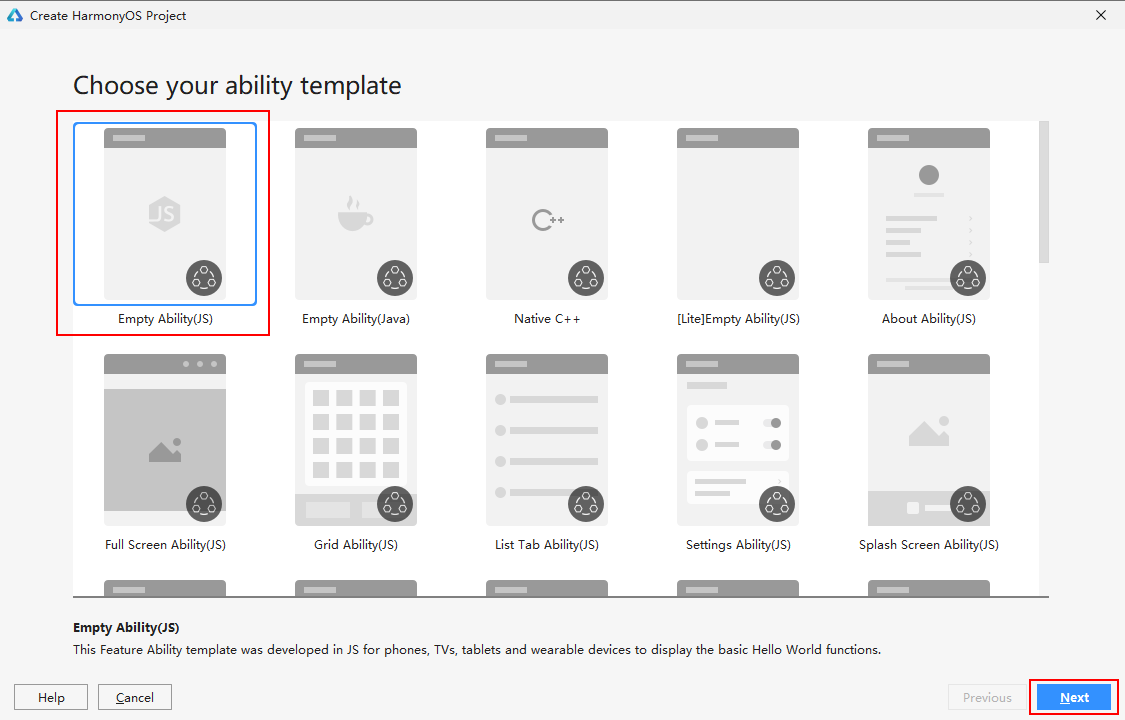配置项目信息